# 机器学习-吴恩达：学习笔记及总结（7）

## Coursera | Machine Learning | Andrew Ng

Thistledown    June 20, 2020

Course Link ：Week7 - Support Vector Machines

## 21 Large Margin Classification

#### 21.1 Optimization Objective

• So far, we’ve seen a range of different algorithms
• With supervised learning algorithms - performance is pretty similar
• What matters often is:
• The amount of training data
• Skill of applying algorithms
• One final supervised learning algorithms that is widely used - Support Vector Machine (SVM)
• Compared to both logistic regression and neural networks, a SVM sometimes gives a cleaner way of learning non-linear functions
• Later in the course we’ll do a survey of different supervised learning algorithms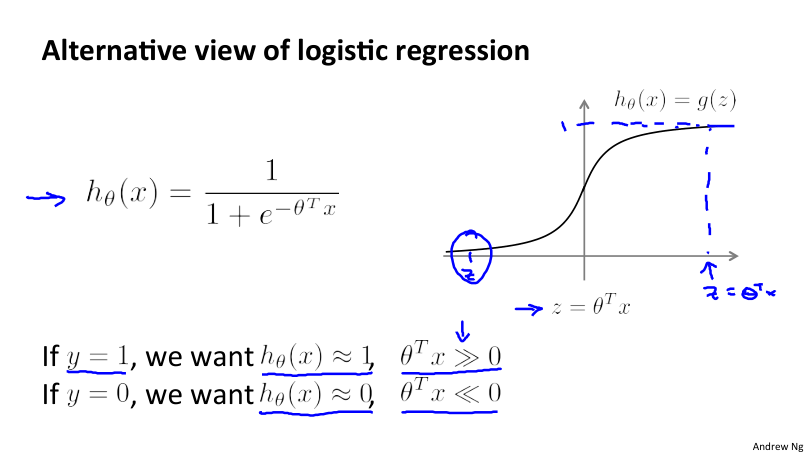• Start with logistic regression, see how we can modify it to get the SVM

• As before, the logistic regression hypothesis is as follows: $h_\theta(x) \frac{1}{1 + e^{-\theta^Tx}}$

• And the sigmoid activation function looks like the one in the picture above.

• In order to explain the math, we use $z$ as defined above

• What do we want logistic regression to do?

• We have an example where $y = 1$
• Then we hope $h_\theta(x)$ is close to 1
• With $h_\theta(x)$ close to 1, $\theta^Tx$ must be much larger than 0
• Similarly, when $y = 0$
• Then we hope $h_\theta(x)$ is close to 0
• With $h_\theta(x)$ close to 0, $\theta^Tx$ must be much less than 0
• This is our classic view of logistic regression, let’s consider another way of thinking about the problem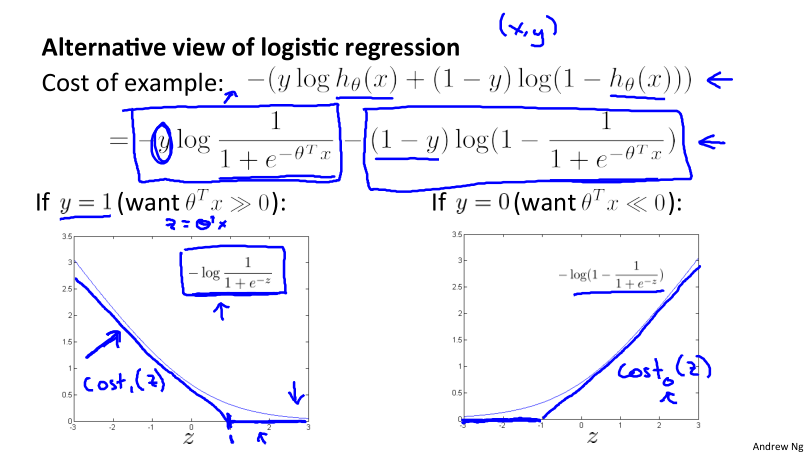• If you look at cost function, each example contributes a term (like the one in the picture) to the overall cost function
• If you then plug in the hypothesis definition $h_\theta(x)$, you get an expanded cost function equation
• So each training example contributes that term to the cost function for logistic regression
• If $y = 1$ then only the first term in the objective matters. The plot of the function vs. $z$ shows that the cost contribution of an example when $y = 1$ given $z$
• So if $z$ is big, the cost is low
• But if $z$ is 0 or negative the cost contribution is high
• This is why, when logistic regression sees a positive example, it tries to set $\theta^Tx$ to be a very large term
• If $y = 0$ then only the second term matters. We can again plot it and get a similar graph. Same deal,
• If $z$ is small then the cost is low
• But if $z$ is large then the cost is massive
• To build a SVM we must define our cost function
• When $y = 1$
• Take the $y = 1$ function and create a new cost function
• Instead of a curved line create two straight lines (magenta) which acts as an approximation to the logistic regression $y = 1$ function
• So this is the new $y=1$ cost function
• Gives the SVM a computational advantage and an easier optimization problem
• We call this function $cost_1(z)$
• Similarly, when $y = 0$
• Do the equivalent with the $y=0$ function plot
• We call this function $cost_0(z)$
• So here we define the two cost function terms for our SVM graphically
• How do we implement this?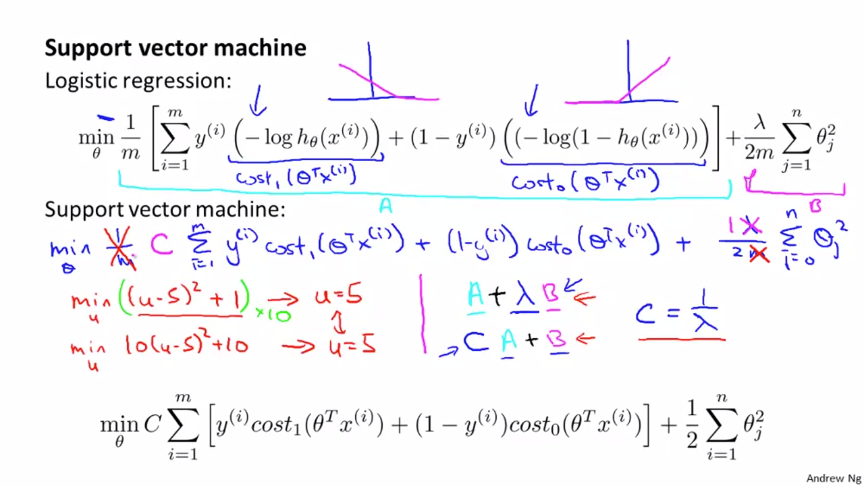• As a comparison/reminder we have logistic regression (as is shown in the picture above)
• For the SVM we take our two logistic regression $y=1$ and $y=0$ terms described previously and replace with $cost_1(\theta^Tx)$ and $cost_0(\theta^Tx)$

• SVM notation is slightly different

#### 21.2 Large Margin Intuition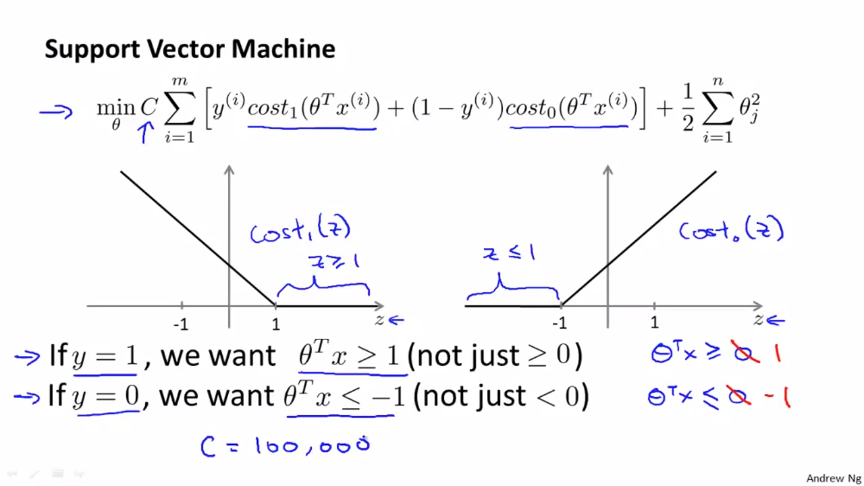• Sometimes people refer to SVM as large margin classifiers
• We’ll consider what that means and what an SVM hypothesis looks like
• The SVM cost function is as above, and we can draw out the cost terms: left is $cost_1$ and right is $cost_0$
• What does it take to make terms small:
• If $y=1$: $cost_1(z)=0$ only when $z \ge 1$
• if $y = 0$: $cost_0(z) = 0$ only when $z \le -1$
• Interesting property of SVM
• In logistic regression, if you have a positive example, you only really need $z$ to be greater or equal to 0
• If this is the case then you predict 1
• SVM wants a bit more than that - doesn’t want to just get it right, but have the value be quite a bit bigger than zero
• Throws in an extra safety margin factor
• Logistic regression dose something similar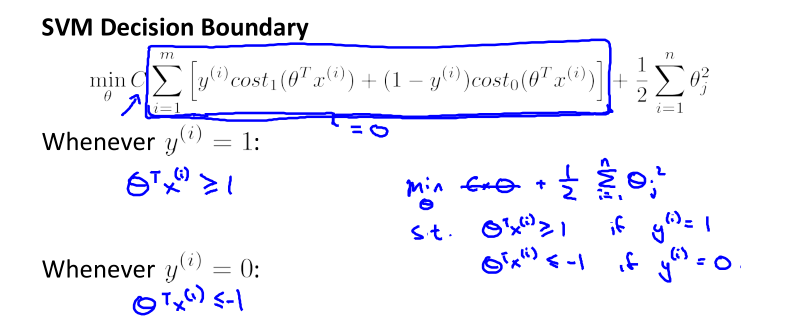• What are the consequences of this? Consider a case where we set C to be huge
• $C = 100000$
• So considering we’re minimizing $CA + B$
• If $C$ is huge we’re going to pick an $A$ value so that $A$ is equal to zero
• What is the optimization problem here - how do we make $A = 0$?
• Making $A = 0$
• If $y = 1$: Then to make our “$A$” term 0 need to find a value of $\theta$ so $(\theta^T x)$ is greater than or equal to 1
• Similarly, if $y = 0$: Then we want to make “$A$” term 0 then we need to find a value of $\theta$ so $(\theta^T x)$ is equal to or less than -1
• So - if we think of our optimization problem a way to ensure that this first “$A$” term is equal to 0, we re-factor our optimization problem into just minimizing the “$B$” (regularization) term, because when $A = 0 \rightarrow A*C = 0$
• So we’re minimizing $B$, under the constraints shown above• Turns out when you solve this problem you get interesting decision boundaries
• The green and magenta lines are functional decision boundaries which could be chosen by logistic regression
• But they probably don’t generalize too well
• The black line, by contrast is the the chosen by the SVM because of this safety net imposed by the optimization graph
• More robust separator
• Mathematically, that black line has a larger minimum distance (margin) from any of the training examples
• By separating with the largest margin you incorporate robustness into your decision making process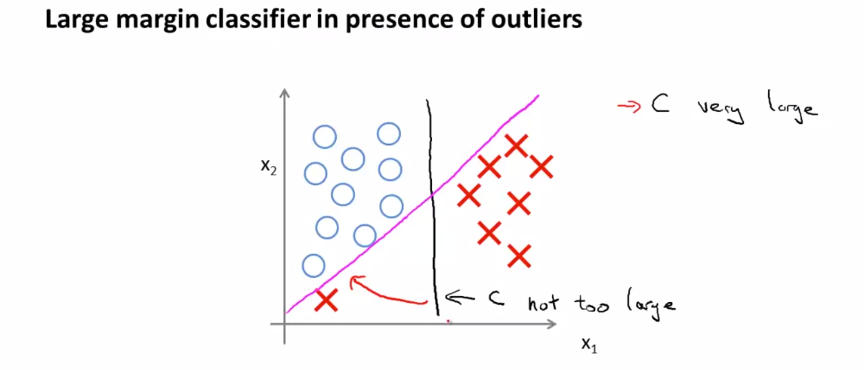• We looked at this at when $C$ is very large
• SVM is more sophisticated than the large margin might look
• If you were just using large margin then SVM would be very sensitive to outliers
• You would risk making a ridiculous hugely impact your classification boundary
• A single example might not represent a good reason to change an algorithm
• If $C$ is very large then we do use this quite naïve maximize the margin approach
• So we’d change the black to the magenta
• What about non-linearly separable data?
• Then SVM still does the right thing if you use a normal size $C$
• So the idea of SVM being a large margin classifier is only really relevant when you have no outliers and you can easily linearly separable data

#### 21.3 Mathematics Behind Large Margin Classification

• Vector inner products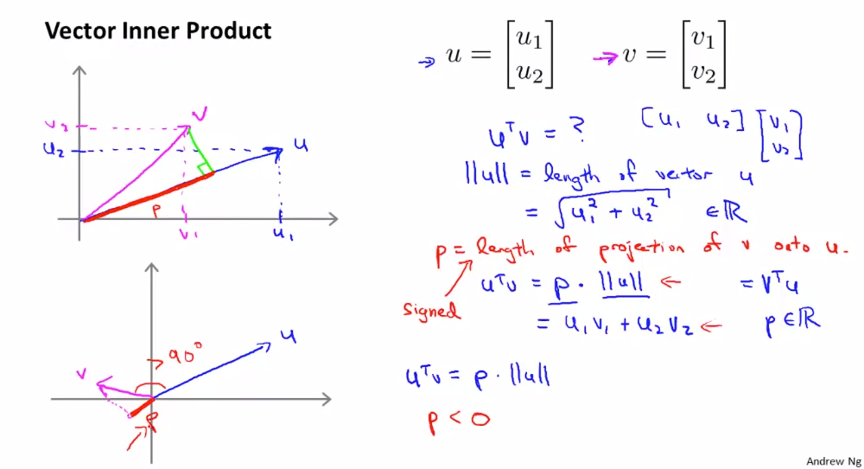• SVM Decision Boundary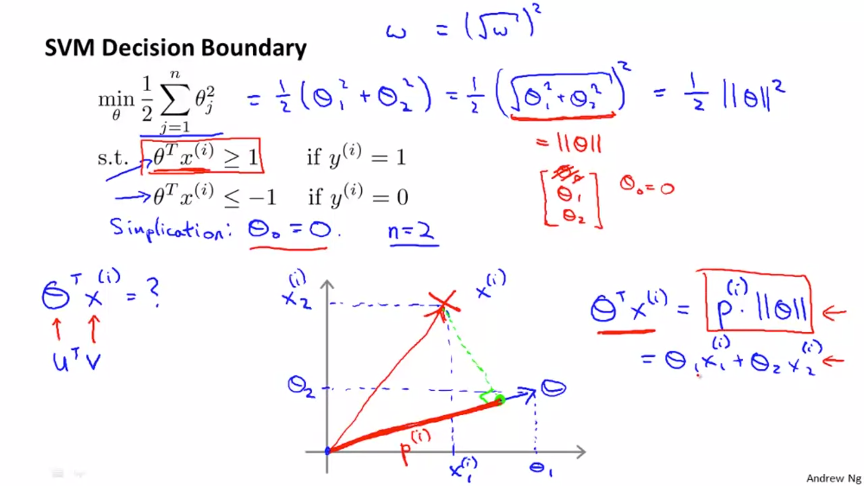• The constraints we defined earlier:

• $\theta^Tx \ge 1 \text{ if } y = 1$
• $\theta^Tx \le -1 \text{ if } y = 0$
• Can be replaced/substituted with the constraints

• $p^i\left|\theta\right| \ge 1 \text{ if } y = 1$
• $p^i\left|\theta\right| \le -1 \text{ if } y = 0$
• Writing that into our optimization objective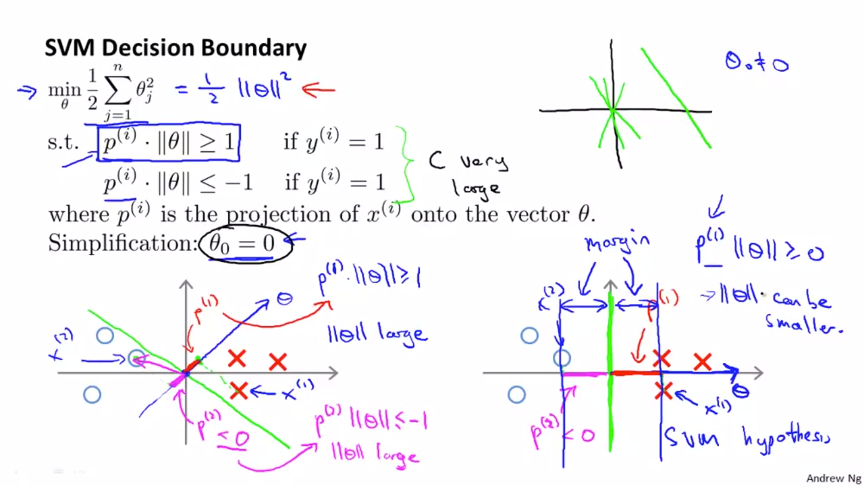## 22 Kernels

#### 22.1 Kernels I

• What are kernels and how do we use them

• We have a training set

• We want to find a non-linear boundary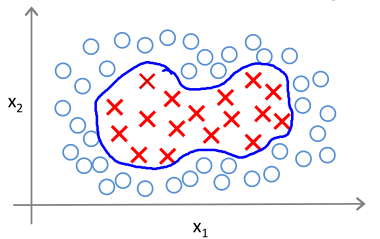• Come up with a complex set of polynomial features to fit the data

• Have $h_\theta(x)$ which
• Returns 1 if the combined weighted sum of vectors (weighted by the parameter vector) is less than or equal to 0
• Else return 0
• Another way of writing this (new notation) is
• That a hypothesis computes a decision boundary by taking the sum of the parameter vector multiplied by a new feature vector $f$, which simply contains the various high order $x$ terms
• e.g., $h_\theta(x) = \theta_0 + \theta_1f_1+\theta_2f_2 + \theta_3 f_3$, where $f_1 = x_1,f_2=x_1x_2,f_3=\cdots$ (i.e. not specific values, but each of the terms from your complex polynomial function)
• Is there a better choice of feature $f$ than the high order polynomials?
• As we saw with computer imaging, high order polynomials become computationally expensive
• New features

• Define three features in this example (ignore $x_0$)

• Have a graph of $x_1$ vs. $x_2$ (don’t plot the values, just define the space)

• Pick three points in that space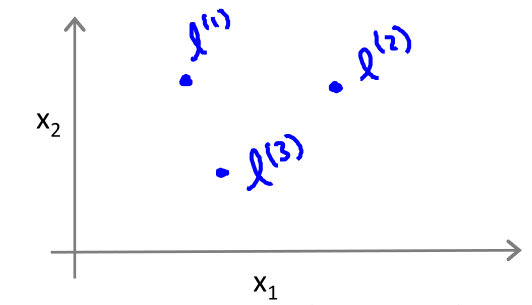• These points $l^1$, $l^2$ and $l^3$, were chosen manually and are called landmarks

• Given $x$, define $f_1$ as the similarity between $(x, l^1)$: $f_1 = \exp(-\frac{|x-l^{(1)}|^2}{2\sigma^2})$
• If we remember our statistics, we know that: $\sigma$ is the standard derivation, $\sigma^2$ is commonly called the variance
• Remember, that as discussed: $|x-l^{(1)}|^2 = \sum_{j =1}^n \left( x_j - l_j^{(1)}\right)^2$
• So, $f_2$ is defined as:

• $f_2 = \text{similarity}(x, l^2) = \exp(-|x-l^{(2)}|^2/{2\sigma^2})$
• And similarly

• $f_3 = \text{similarity}(x, l^3) = \exp(-|x-l^{(3)}|^2/{2\sigma^2})$
• This similarity function is called a kernel

• The function is a Gaussian Kernel
• So, instead of writing similarity between $x$ and $l$ we might write $f_1 = k(x, l^1)$

• Driving deeper into the kernel

• So lets see what these kernels do and why the functions defined make sense
• Say $x$ is close to a landmark,
• Then the squared distance will be $\sim0$
• So $f_1 \approx \exp(-0^2/2\sigma^2) \approx e^{-0} \approx 1$
• Say $x$ is far from a landmark
• Then the squared distance is big
• Gives $e^{\text{- large number}} \approx 0$
• Each landmark defines a new features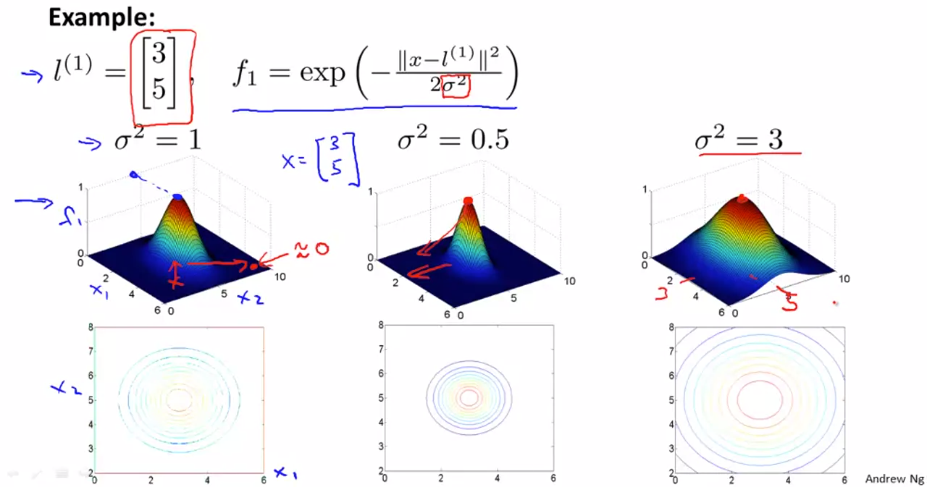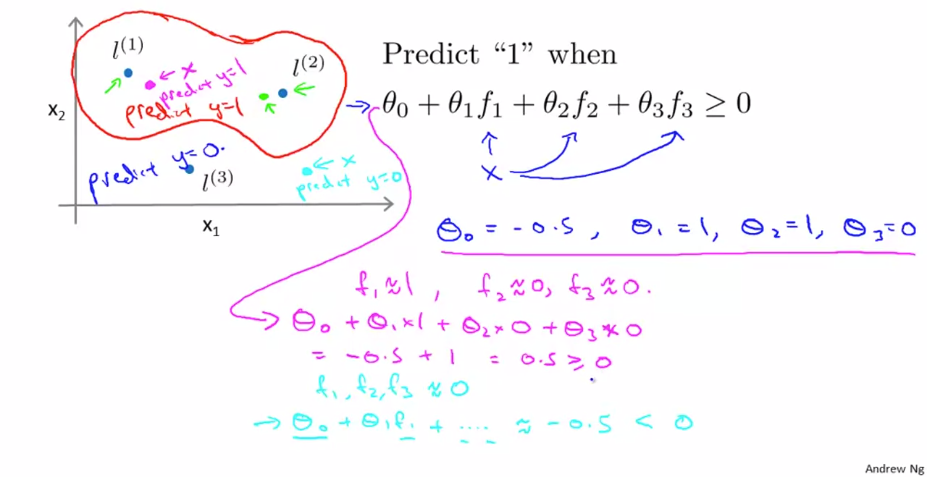#### 22.2 Kernels II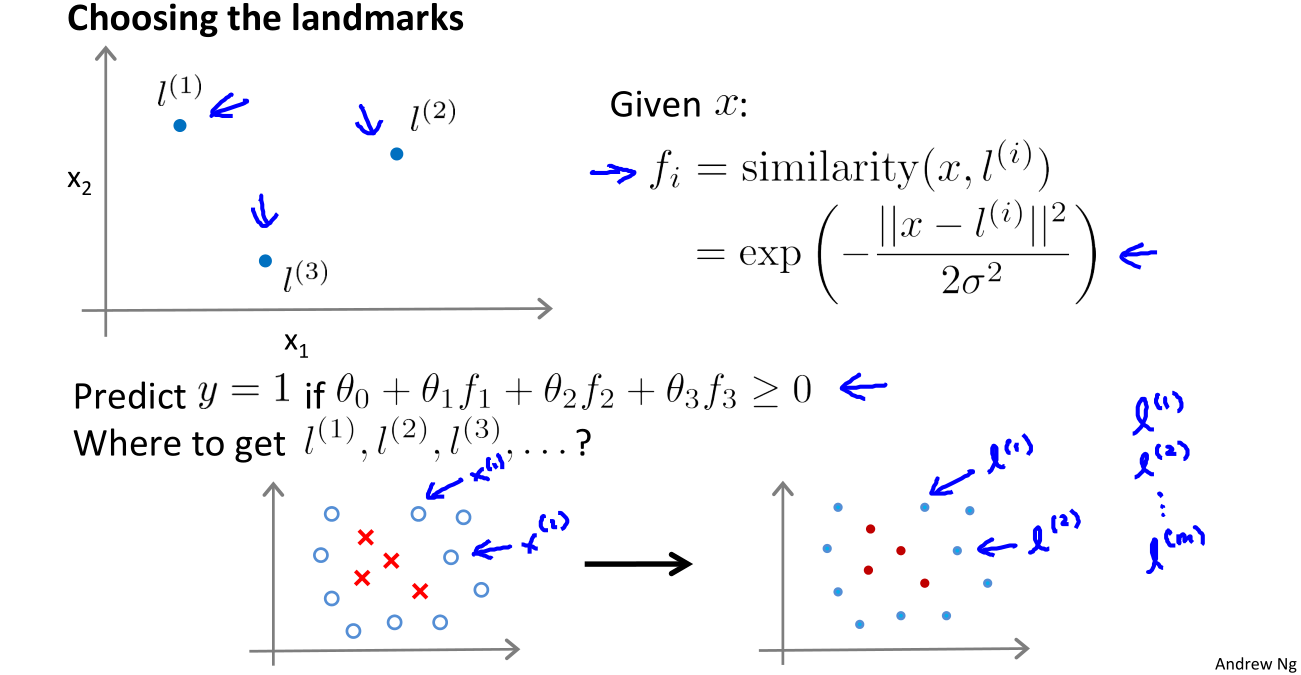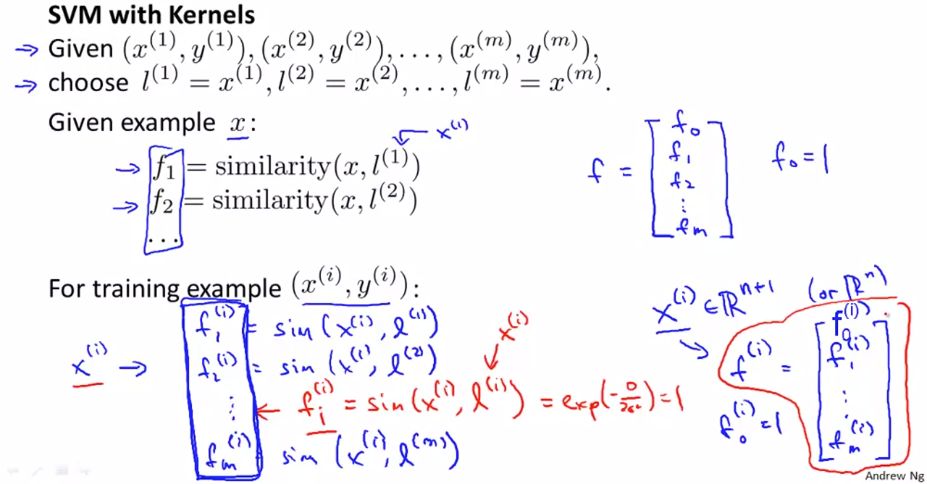• Take the training data

• For each example place a landmark at exactly the same location

• So end up with $m$ landmarks

• One landmark per location per training example
• Means our features measure how close to a training set example something is
• Given a new example, compute all the f values

• Gives you a feature vector $f$ ($f_0 \cdots f_m$)
• $f_0 = 1$ always
• A more detailed look at generating the f vector

• If we had a training example - features we compute would be using $(x_i, y_i)$

• So we just cycle through each landmark, calculating how close to that landmark actually $x_i$ is
• $f_1^i, = k(x^i, l^1)$
• $f_2^i, = k(x^i, l^2)$
• $…$
• $f_m^i, = k(x^i, l^m)$
• Somewhere in the list we compare $x$ to itself… (i.e. when we’re at $f_i^i$)
• So because we’re using the Gaussian Kernel this evaluates to 1
• Take these $m$ features $(f_1, f_2 \cdots f_m)$ group them into an $[m +1 \times 1]$ dimensional vector called $f$
• fi is the f feature vector for the $i^{\text{th}}$ example
• And add a $0^{\text{th}}$ term = 1
• Given these kernels, how do we use a support vector machine

• SVM hypothesis prediction with kernels

• Predict $y = 1$ if $\theta^Tf \ge 0$
• Because $\theta = [m + 1 \times 1]$, $f = [m + 1 \times 1]$
• So, this is how you make a prediction assuming you already have $\theta$
• How do you get $\theta$?
• SVM training with kernels

• Using the SVM learning algorithm $\mathop{\text{min}}_\limits{\theta} C \sum_{i=1}^m y^{(i)}cost_1(\theta^Tf^{(i)}) + (1 - y^{(i)})cost_0(\theta^Tf^{(i)}) + \frac{1}{2}\sum_{j=1}^{n} \theta^2_j$

• Now, we minimizing using $f$ as the feature vector instead of $x$
• By solving this minimization problem you get the parameters for your SVM
• In this setup, $m = n$

• Because number of features is the number of training data examples we have
• One final mathematic detail (not crucial to understand)

• If we ignore $\theta_0$ then $\sum_{j = 1}^n \theta^2_j = \theta^T\theta$ is true
• What many implementations do is $\theta^TM\theta$
• Where the matrix $M$ depends on the kernel you use
• Gives a slightly different minimization - means we determine a rescaled version of $\theta$
• Allows more efficient computation, and scale to much bigger training sets
• If you have a training set with 10,000 values, means you get 10,000 features
• Solving for all these parameters can become expensive
• So by adding this in we avoid a for loop and use a matrix multiplication algorithm instead
• You can apply kernels to other algorithms

• But they tend to be very computationally expensive
• But the SVM is far more efficient - so more practical
• Lots of good off the shelf software to minimize this function, you don’t need to write your own

• SVM parameters ($C$)

• Bias and variance trade off
• Must chose $C$:
• $C$ plays a role similar to $1/\lambda$ (where $\lambda$ is the regularization parameter)
• Large $C$ gives a hypothesis of low bias & high variance → overfitting
• Small $C$ gives a hypothesis of high bias & low variance → underfitting
• SVM parameters ($\sigma^2$)

• Parameters for calculating $f$ values
• Large $\sigma^2$ → $f$ features vary more smoothly → higher bias, low variance
• Small $\sigma^2$ → $f$ features vary more abruptly → lower bias, higher variance

## 23 SVMs in Practice

#### 23.1 Using An SVM

• Choosing a kernel
• We’ve looked at the Gaussian kernel
• Need to define $\sigma$ ($\sigma^2$)
• When would you chose a Gaussian?
• If $n$ is small and/or $m$ is large
• If you’re using a Gaussian kernel then you may need to implement the kernel function
• Note: make sure you perform feature scaling before using a Gaussian kernel
• Could use no kernel - linear kernel
• Predict $y = 1$ if $\theta^Tx \ge 0$
• So no $f$ vector
• Get a standard linear classifier
• Why do this?
• If $n$ is large and $m$ is small then
• Lots of features, few examples
• Not enough data - risk overfitting in a high dimensional feature-space
• Other choice of kernel
• Linear and Gaussian are most common
• Not all similarity functions are valid kernels
• Must satisfy Mercer's Theorem
• SVM use numerical optimization tricks
• Mean certain optimization can be made, but they must follow the theorem
• Polynomial Kernel: $(x^Tl + \text{const})^{\text{degree}}$
• Usually performs worse than the Gaussian kernel
• Used when $x$ and $l$ are both non-negative
• String kernel
• Used if input is text strings
• Use for text classification
• Chi-squared kernel
• Histogram intersection kernel
• Multi-class classification for SVM
• Many packages have built in multi-class classification packages
• Otherwise use one-vs all method
• Logistic regression vs. SVM
• When should you use SVM and when is logistic regression more applicable
• If $n$ (features) is large vs. $m$ (training set) is small: Then use logistic regression or SVM with a linear kernel
• If $n$ is small and $m$ is intermediate: Gaussian kernel is good
• If $n$ is small and $m$ is large: SVM will be slow to run with Gaussian kernel
• Manually create or add more features
• Use logistic regression of SVM with a linear kernel
• Logistic regression and SVM with a linear kernel are pretty similar
• Do similar things
• Get similar performance
• A lot of SVM’s power is using different kernels to learn complex non-linear functions
• For all these regimes a well designed NN should work
• But, for some of these problems a NN might be slower - SVM well implemented would be faster
• SVM has a convex optimization problem - so you get a global minimum
• It’s not always clear how to chose an algorithm
• Often more important to get enough data
• Designing new features
• Debugging the algorithm
• SVM is widely perceived a very powerful learning algorithm

## Ex6: Support Vector Machines👨‍💻

See this exercise on Coursera-MachineLearning-Python/ex6/ex6.ipynb

Thistledown